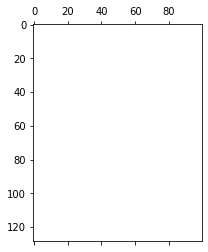# Spectrogram by torch inconsistent with scipy.signal

I’m trying to replace `scipy.signal` with `torch` for audio preprocessing (and I don’t want to use `torchaudio` because I need to export the whole pipeline to C++).
So, I need log-spectrogram, which is S = log(|STFT(x)|^2), but I get unexpected shapes from pytorch.

Minimal code to reproduce:

``````from scipy import signal
import torch

data = np.random.uniform(-1, 1, 22500)
n_fft = 256

s, t, z = signal.spectrogram(data, nperseg=n_fft)
ss = torch.stft(torch.Tensor(data), n_fft)

print(z.shape)  # (129, 100)
print(ss.shape) # torch.Size([129, 352, 2])
``````

You can see that the shapes do not match, and the output of `torch` has a lot of infs/nans:
`plt.spy(z) `:`plt.spy(np.log(ss[:,:, 0]*ss[:, :, 1]))`:How can I properly compute the spectrogram using torch only?